# How is the strength of a magnet tested?

Shop for MagnetsThe strength of a magnet is indicated by either its pull force or its magnetic field strength. A magnet’s strength is always stated in the product information for a magnet, however manufacturers use the following methods to discern this:

## Pull force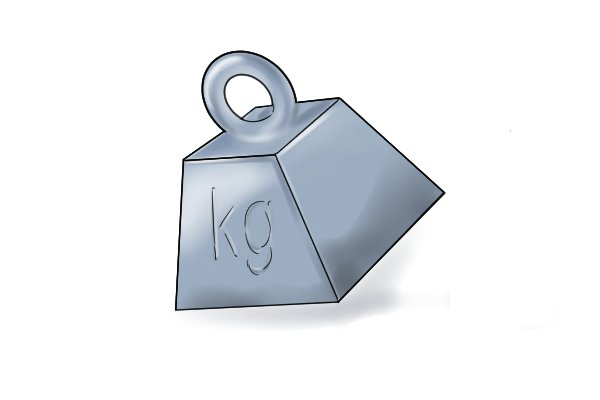The pull force of a magnet is the amount of force required to pull a magnet away from the ferromagnetic material it is attached to. The pull force of a magnet is measured in either kilogrammes (kg) or newtons (N) by a pull force tester.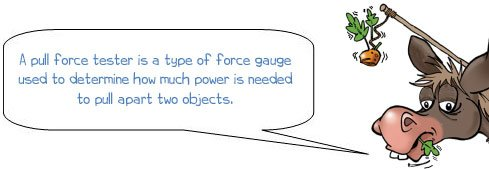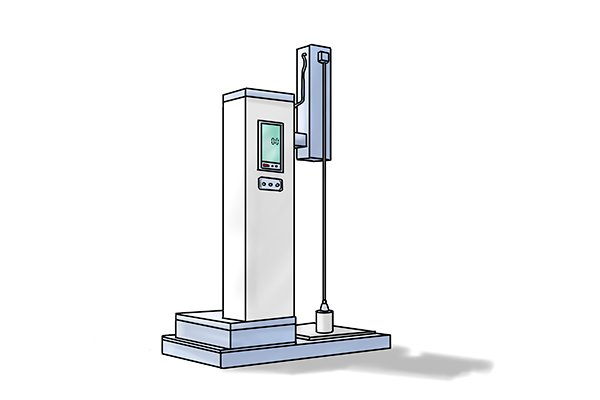To use a pull force tester, a magnet is placed onto a thin steel sheet and the amount of force it takes to pull the magnet away from the steel is then measured.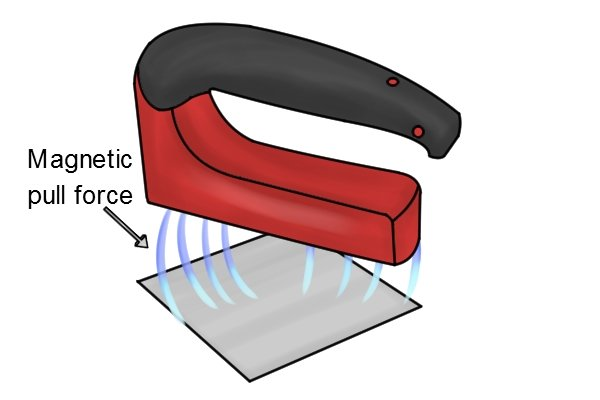The pull force of a magnet is determined by the strength of the magnetic force and not by its overall size. This means that a large magnet does not necessarily have a larger pull force compared with a small magnet.

## Magnetic field strengthThe magnetic field strength of a magnet is measured in two units of measurement, discovered by Nikola Tesla and Carl Friedrich Gauss, called gauss and tesla.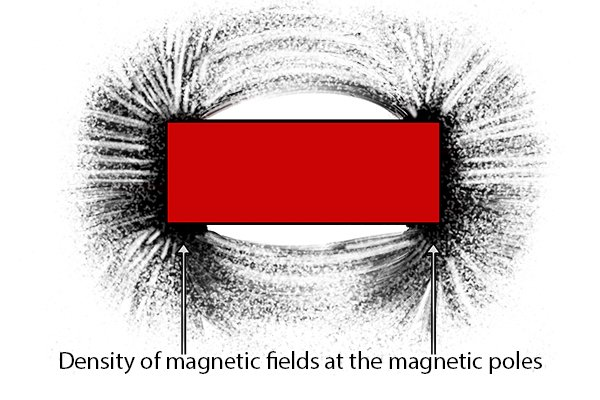Gauss and tesla both measure how dense the magnetic field is on the surface of a magnet. This is also known as a magnet’s ‘magnetic induction’.

To measure the magnetic field strength more accurately, the measurement ‘gauss’ is used. This is because 10,000 gauss are equal to one tesla.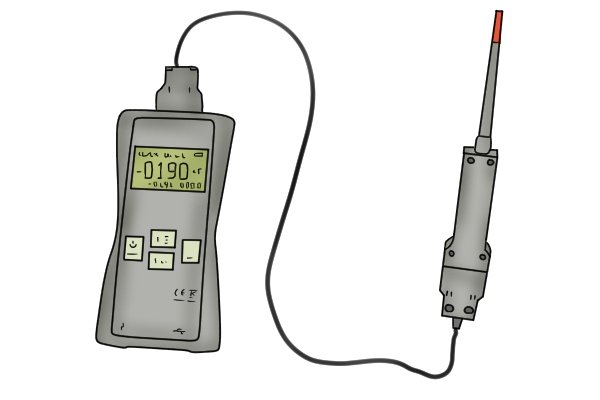The magnetic induction of a magnet is measured by a gauss-meter. A gauss meter works according to the hall effect, which is where electrical current flows through, and builds up to make itself equal to, a magnetic field. This build-up produces a readable voltage, which the gauss meter detects.

Call Now Button